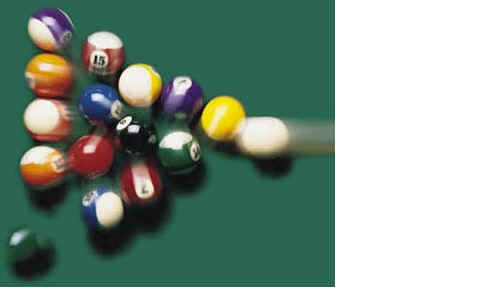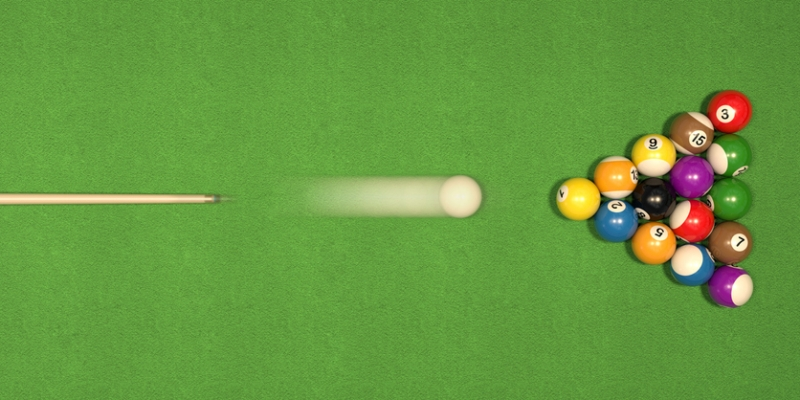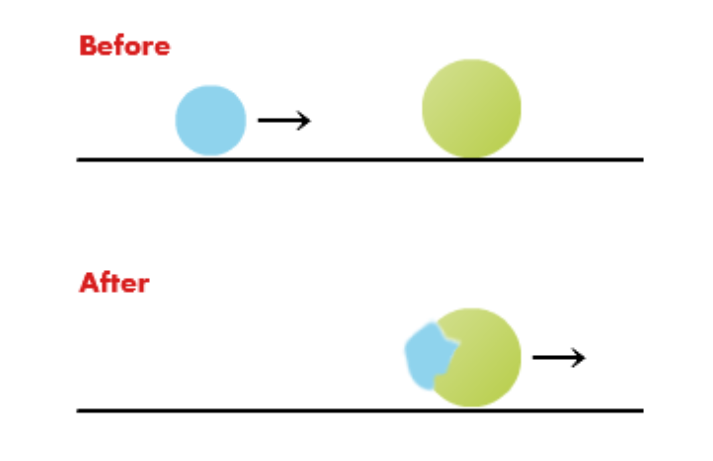# Collision Study Guide

Collisions are a common occurrence every day. However, the effects can be different in elastic and inelastic collisions.

# INTRODUCTION

How often did you think you were going to sink the ball this time for sure, only to have it fly off in unexpected directions? It takes a while for any beginner to get the hang of billiards and get the direction right. To master the game, you must understand impulse force, momentum, and collisions. When you strike the cue ball in the pool, you can observe how the ball's kinetic energy splits up into the other balls to set them in motion. What are the physics principles behind billiards? Let’s find out!Source

## WHAT IS A COLLISION?

In physics, a collision refers to objects in motion that come in contact with each other such that they exert a mutual impulsive force. Collisions involve the transfer of kinetic energy from one body to another. Based on that, collisions can be classified as:

Collision in physics refers to objects in motion coming in contact such that they exert an impulsive force on one another. There is a transfer of kinetic energy between the colliding objects in collisions. In a closed system (a system that doesn’t exchange heat, work, or matter to the surroundings), the momentum is conserved.

Some collisions in a closed system occur such that the kinetic energy is also conserved. Such collisions are called elastic collisions. If any denting, deformation, or elevation against the gravity of the objects involved in a collision, then the kinetic energy is not conserved. Such collisions are called inelastic collisions.

## ELASTIC COLLISIONSource

In an elastic collision, the net kinetic energy of the objects before and after the collision remains the same. In other words, kinetic energy is transferred between the two bodies without being lost.

Considering the billiard balls example, the kinetic energy of the cue ball before the collision is almost equal to the sum of kinetic energy gained by all other balls after the collision. Because the balls are of the same mass and made out of a hard material that doesn’t deform under the forces of the pool, the collision of billiard balls can be considered nearly elastic.

## INELASTIC COLLISIONSource

Inelastic collisions experience a loss of kinetic energy as the energy is transformed into heat, sound, or physical deformation of materials. Most collisions in the real world are inelastic. Observe the deformation of the golf ball in the picture as it collides with a club. During the collision, the golf ball compresses and expands and, in the process, loses kinetic energy in the form of sound and heat.

## MOMENTUM AND IMPULSE

Momentum gives you a measure of how much motion exists in a moving object. A slow-moving bowling ball has lesser momentum than a fast-moving bowling ball. Mathematically momentum is expressed as:

``````                           p = mv
``````

p is momentum in kg m/s

m is the mass of the object in kg

v is the velocity of the object in m/s

An impulse is a force that acts for a certain period, bringing about a change in momentum. Examples of an impulse include kicking a ball, striking with a bat, and breaking boards in martial arts. Mathematically it is expressed as force multiplied by time:

``````                           J = Ft
``````

J is the impulse in Ns F is the force in Newton t is the time duration in seconds

## CONSERVATION OF MOMENTUMSource

According to the law of conservation of momentum, in an isolated system, the total momentum of the colliding objects remains the same before and after the collision. That means the momentum gained by one object after the collision is equal to the momentum lost by the other. This is the reason why guns recoil when fired. According to Newton’s 3rd law, shooting the bullet in one direction creates an equal and opposite reaction as the recoil. The momentum of the gun's recoil is equal to the bullet's momentum. Mathematically it is expressed as:

``````                           MgVg = - MbVb
``````

Mg = mass of the gun

Vg = velocity of the gun

Mb = mass of the bullet

Vb = velocity of the bullet

# CONCLUSION:

• A collision occurs when two bodies come in direct contact and exert force on each other.
• In an elastic collision, there is no net loss of kinetic energy
• In inelastic collisions, there is a loss of kinetic energy
• According to the law of conservation of momentum, in an isolated system, the total momentum of the colliding objects remains the same before and after the collision.

## FAQs:

1. What is a synonym for collision?

Going by the collision definition, it can also be called an impact or an impulse.

2. What are the 3 types of the collision?

• Elastic
• Inelastic
• Completely inelastic

3. What happens in a collision?

There is the transfer of kinetic energy during a collision, but the system's total momentum remains the same.

We hope you enjoyed studying this lesson and learned something cool about Collision! Join our Discord community to get any questions you may have answered and to engage with other students just like you! We promise, it makes studying much more fun! 😎

## SOURCES:

1. 6.4 Elastic and Inelastic Collisions. https://flexbooks.ck12.org/cbook/ck-12-physics-flexbook-2.0/section/6.4/related/lecture/elastic-and-inelastic-collisions/. Accessed 27 Jan 2022.
2. 6.1 Momentum and Impulse. https://flexbooks.ck12.org/cbook/ck-12-physics-flexbook-2.0/section/6.1/primary/lesson/momentum-and-impulse-phys/. Accessed 27 Jan 2022.
3. Elastic Collision. https://byjus.com/physics/elastic-collision/. Accessed 27 Jan 2022.
4. What are elastic and inelastic collisions?. https://www.khanacademy.org/science/physics/linear-momentum/elastic-and-inelastic-collisions/a/what-are-elastic-and-inelastic-collisions. Accessed 27 Jan 2022.